Command Reference : Matrix Language Reference

 @commute
Commutation matrix.
Syntax: @commute(m, n)
m: integer
n: integer
Return: matrix
The commutation matrix transforms the vectorization of a matrix to the vectorization of its transpose.
Given the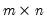matrix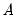, returns the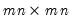matrix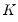, which satisfies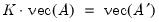Examples
matrix m1 = @mnrnd(10, 5)
vector diff = @commute(10, 5) * @vec(m1) - @vec(m1.@t)
demonstrates the properties of the commutation matrix since DIFF equals zero.
Cross-references